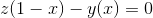### # Methodology

First, we need to understand how vertical spreads work. To create a vertical spread, a person goes long on a strike and short on another strike with the same expiration. For this walkthrough, we will focus on selling options for simplicity.

For a person to receive a credit on selling a vertical spread, they would sell the strike closer to the money, and purchase a strike that is further away from the money. This is because the strike closer to the money has a higher value than the other strike, therefore yielding a credit.

A tight vertical spread refers to vertical spreads with strike prices that are closer together (we use strikes that are a dollar apart to calculate our data, generally). Tight vertical spreads act more like our tickets from the getting started analogy because there is a smaller chance of the stock price ending up between the two strikes, which would cause error in our calculations.

Our data model uses a conservative approach - we assume that we take on the maximum loss when the stock price passes the short strike of our spread.

### # Example

Let's take a quick look at a small excerpt of data provided by our system:From the data, we observe the following:

• The current price of SPLK is \$129.60
• By selling a 135 - 136 vertical spread using calls, we would get 0.25 cents and by risking a total of 0.75 cents.
• The price of SPLK needs to rise 4.17%, or above 135, for us to incur a maximum loss.

The following formula comes into play:In this case, z equals the credit, y equals the maximum risk, and x equals the chance that the stock price will go past our short strike of 135. The expected outcome of this formula is 0 because in a perfectly priced marketplace, neither buyers or sellers of the spread would earn money, given enough repetitions.

By solving this formula, we see that the market believes there is a 25% chance of the trade being a loser (rising more than 4.17%). This is our evZeroVal.

### # Historical Probability Comparison

Now that we know that the market believes that there is only a 25% chance of SPLK rising more than 4.17% in the next earnings event, we can compare this to the historical moves of SPLK after earnings.

Our database shows us that in the last 28 SPLK earnings events, SPLK has risen above 4.17% 11 times. This gives us the actual probability of the rise at nearly 40%, which is much greater than what the market is currently predicting.

By dividing the evZeroVal by the actual probability, we now have the edge value.

### # Calculations

Let's say we theoretically sold each spread during previous 28 SPLK earnings:

#### # Selling for credit with favorable edge

By selling the 125 - 124 spread with an edge of 1.73, we would have 6 losing trades. Our total profit after all the earnings would be: 0.37 x 22 - 0.63 x 6 = 4.36 total P/L.

#### # Selling for credit with unfavorable edge

By selling the 135 - 136 spread with an edge of 0.64, we would have 11 losing trades. Our total profit after all the earnings would be: 0.25 x 17 - 0.75 x 11 = -4.00 total P/L.

#### # Selling for credit with fair edge

By selling the 127 - 126 spread with an edge of 1.02, we would have 11 losing trades. Our total profit after all the earnings would be: 0.4 x 17 - 0.6 x 11 = 0.20 total P/L.

As we can see, the edge is a powerful tool that allows us to see where the market both overprices and underprices risk.

We have only scratched the surface of what EarningsViz can offer. Learn more by reading our tips and tricks